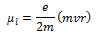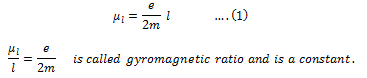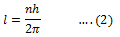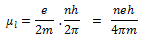According to Neil Bohr’s atom model, the negatively charged electron is revolving around a positively charged nucleus in a circular orbit of radius r. The revolving electron in a closed path constitutes an electric current. The motion of the electron in anticlockwise direction produces conventional current in the clockwise direction.

Current, I = e/T where T is the period of revolution of the electron.

If v is the orbital velocity of the electron, thenDue to the orbital motion of the electron, there will be orbital magnetic moment μlIf m is the mass of the electronmvr is the angular momentum (l) of the electron about the central nucleus.Its value is 8.8 × 1010 C kg-1. Bohr hypothesized that the angular momentum has only discrete set of values given by the equation.where n is a natural number and h is the Planck’s constant = 6.626 × 10-34 Js.

Substituting equation (2) in equation (1)The minimum value of magnetic moment isThe value of eh/4πm is called Bohr magneton.

By substituting the values of e, h and m, the value of Bohr magneton is found to be 9.27 × 10–24 Am2

In addition to the magnetic moment due to its orbital motion, the electron possesses magnetic moment due to its spin. Hence the resultant magnetic moment of an electron is the vector sum of its orbital magnetic moment and its spin magnetic moment.